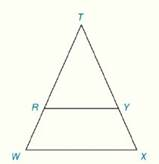Chapter 4.CR, Problem 30CR### Elementary Geometry for College St...

6th Edition
Daniel C. Alexander + 1 other
ISBN: 9781285195698

#### Solutions

Chapter
Section### Elementary Geometry for College St...

6th Edition
Daniel C. Alexander + 1 other
ISBN: 9781285195698
Textbook Problem
1 views

# Review Exercises Given: ∆ T W X is an isosceles, with base W X - R Y - ∥ W X - Prove: R W X Y is an isoscels trapezoidTo determine

To Prove:

The quadrilateral RWXY mube be an isosceles trapezoid.

Explanation

Proof:

Definition:

A trapezoid is a quadrilateral with exactly two parallel sides.

If the two legs of a trapezoid are congruent, the trapezoid is known as an isosceles trapezoid.

Description:

It is given that TWX is an isosceles, with base WX¯ and RY¯WX¯.

Since RY¯WX¯, quadrilateral RWXY must be a trapezoid by its definition.

As the TWX is an isosceles with base WX¯, its sides must be in equal measure

### Still sussing out bartleby?

Check out a sample textbook solution.

See a sample solution

#### The Solution to Your Study Problems

Bartleby provides explanations to thousands of textbook problems written by our experts, many with advanced degrees!

Get Started

#### In Problems 1-12, find the derivative of each function. 4.

Mathematical Applications for the Management, Life, and Social Sciences

#### Prove that limx21x=12.

Single Variable Calculus: Early Transcendentals, Volume I

#### In Exercises 2336, find the domain of the function. 28. f(x)=x5

Applied Calculus for the Managerial, Life, and Social Sciences: A Brief Approach

#### The directrix of the conic given by r=62+10sin is: a) x=53 b) x=35 c) y=53 d) y=35

Study Guide for Stewart's Single Variable Calculus: Early Transcendentals, 8th